Logarithmic Differentiation - Type 2

Chapter 5 Class 12 Continuity and Differentiability
Concept wise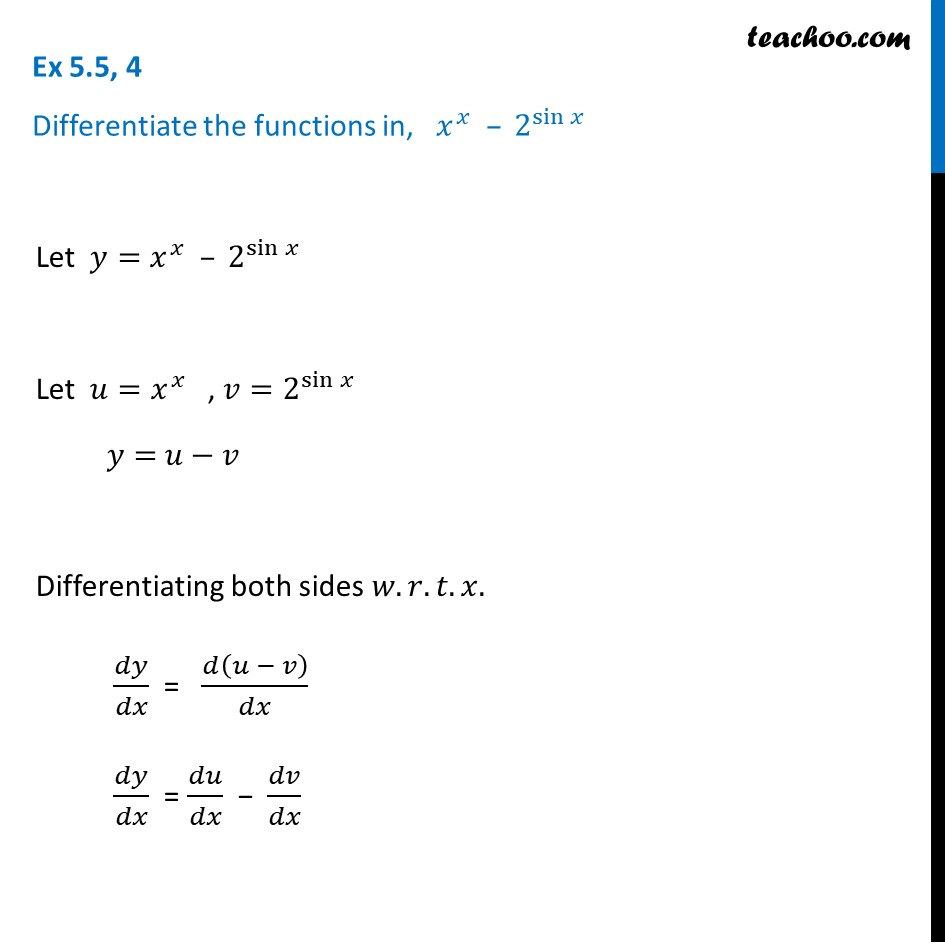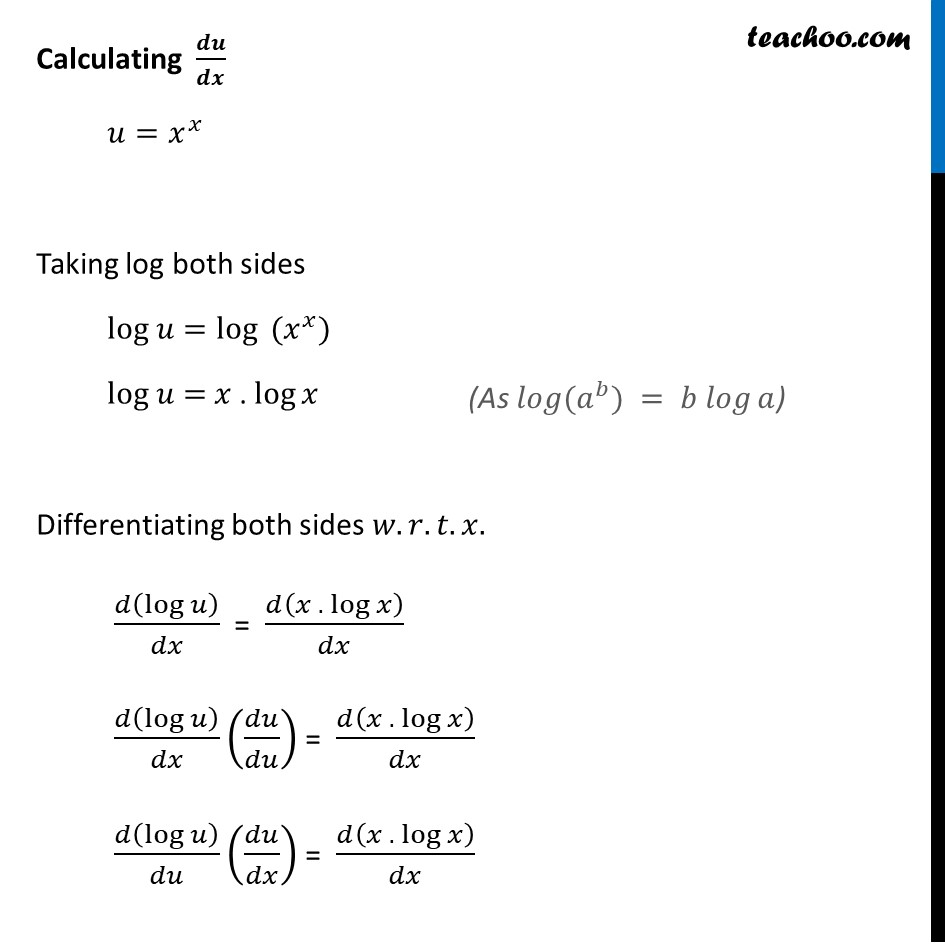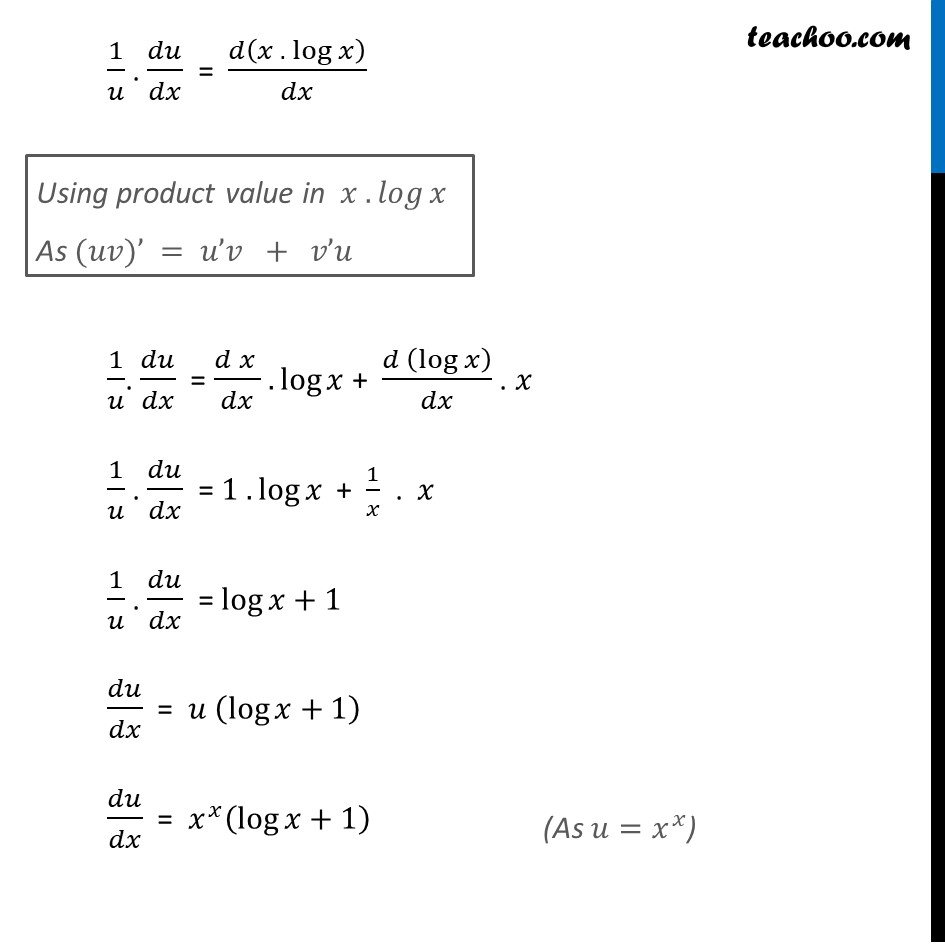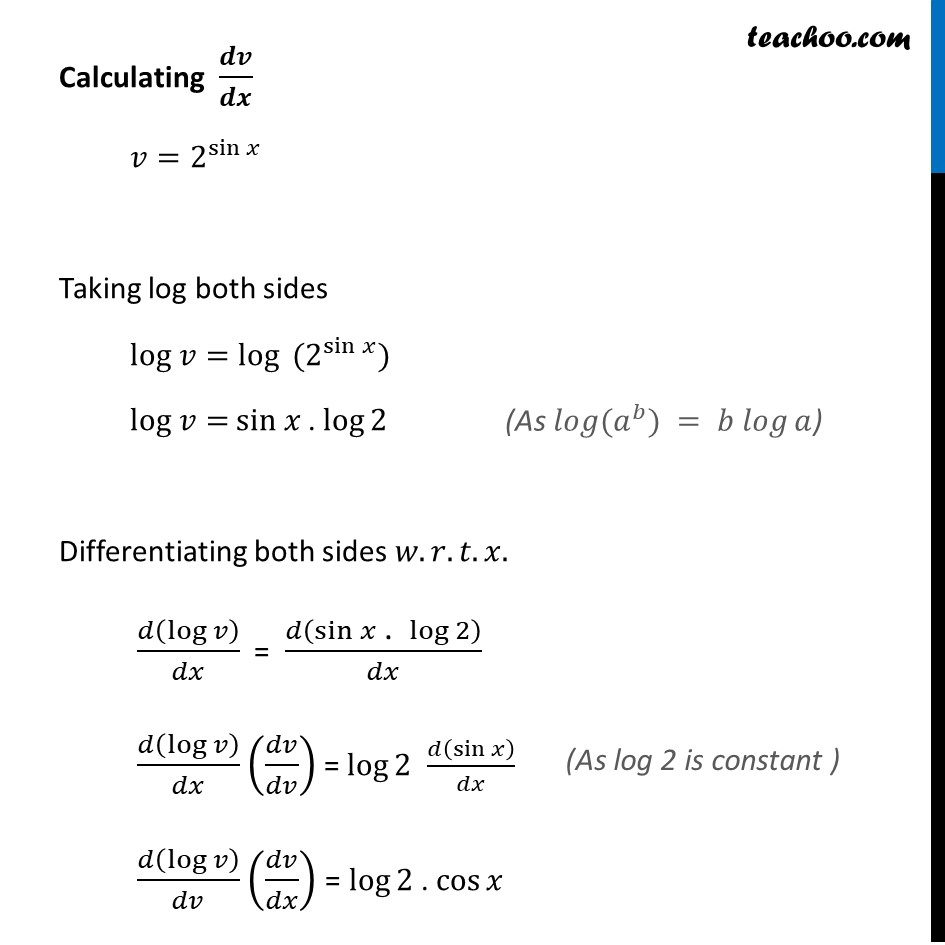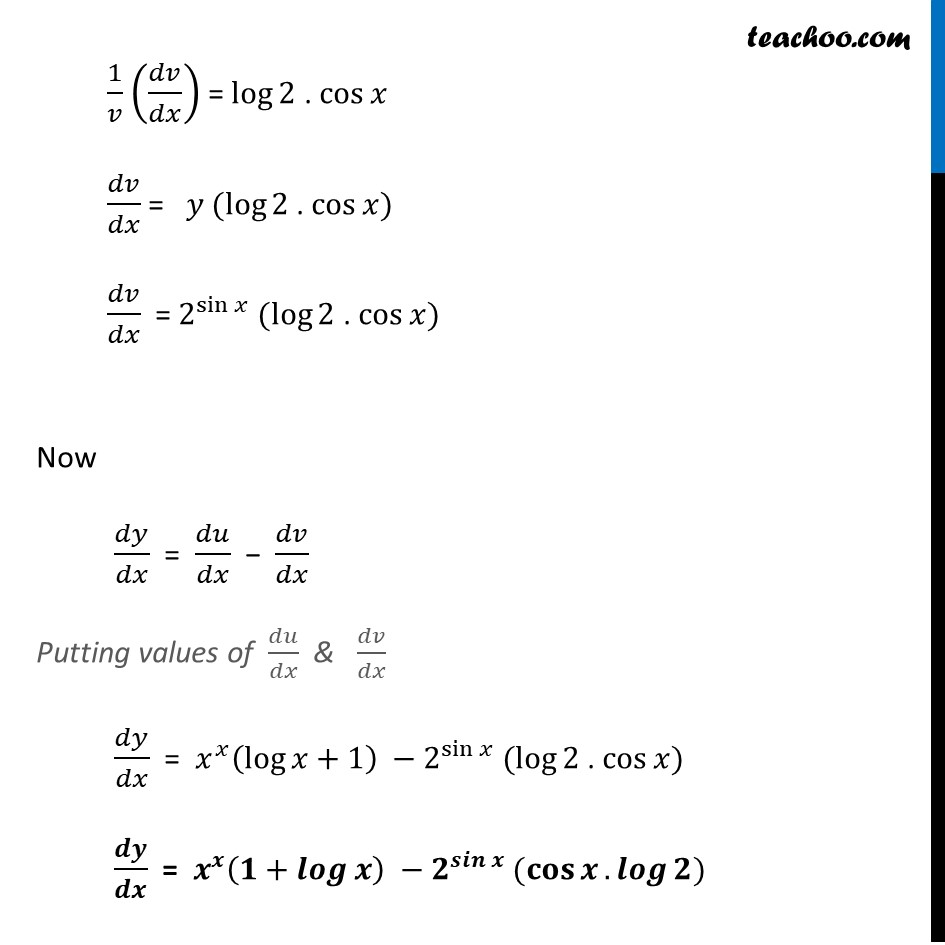Introducing your new favourite teacher - Teachoo Black, at only ₹83 per month

### Transcript

Ex 5.5, 4 Differentiate the functions in, 𝑥^𝑥 – 2^sin⁡𝑥 Let 𝑦=𝑥^𝑥 – 2^sin⁡𝑥 Let 𝑢=𝑥^𝑥 , 𝑣=2^sin⁡𝑥 𝑦= 𝑢−𝑣 Differentiating both sides 𝑤.𝑟.𝑡.𝑥. 𝑑𝑦/𝑑𝑥 = 𝑑(𝑢 − 𝑣)/𝑑𝑥 𝑑𝑦/𝑑𝑥 = 𝑑𝑢/𝑑𝑥 − 𝑑𝑣/𝑑𝑥 Calculating 𝒅𝒖/𝒅𝒙 𝑢=𝑥^𝑥 Taking log both sides log 𝑢=〖log⁡〖 (𝑥〗〗^𝑥) log 𝑢= 𝑥 . log⁡𝑥 Differentiating both sides 𝑤.𝑟.𝑡.𝑥. 𝑑(log⁡𝑢 )/𝑑𝑥 = 𝑑(𝑥 . log⁡𝑥 )/𝑑𝑥 𝑑(log⁡𝑢 )/𝑑𝑥 (𝑑𝑢/𝑑𝑢) = 𝑑(𝑥 . log⁡𝑥 )/𝑑𝑥 𝑑(log⁡𝑢 )/𝑑𝑢 (𝑑𝑢/𝑑𝑥) = 𝑑(𝑥 . log⁡𝑥 )/𝑑𝑥 v(As 𝑙𝑜𝑔⁡(𝑎^𝑏) = 𝑏 𝑙𝑜𝑔⁡𝑎) 1/𝑢 . 𝑑𝑢/𝑑𝑥 = 𝑑(𝑥 . log⁡𝑥 )/𝑑𝑥 1/𝑢. 𝑑𝑢/𝑑𝑥 = (𝑑 𝑥 )/𝑑𝑥 .log⁡𝑥 + (𝑑 (log⁡𝑥 ))/𝑑𝑥 . 𝑥 1/𝑢 . 𝑑𝑢/𝑑𝑥 = 1 . log⁡𝑥 + 1/𝑥 . 𝑥 1/𝑢 . 𝑑𝑢/𝑑𝑥 = log⁡〖𝑥+1〗 𝑑𝑢/𝑑𝑥 = 𝑢 (log⁡〖𝑥+1〗 ) 𝑑𝑢/𝑑𝑥 = 𝑥^𝑥 (log⁡〖𝑥+1〗 ) Using product value in 〖𝑥 .〗⁡𝑙𝑜𝑔⁡𝑥 As (𝑢𝑣)’ = 𝑢’𝑣 + 𝑣’𝑢 Calculating 𝒅𝒗/𝒅𝒙 𝑣=2^sin⁡𝑥 Taking log both sides log 𝑣=log⁡〖 (2^sin⁡𝑥 ) " " 〗 log 𝑣= sin 𝑥 . log⁡2 Differentiating both sides 𝑤.𝑟.𝑡.𝑥. 𝑑(log⁡𝑣 )/𝑑𝑥 = 𝑑(sin 𝑥" . " log⁡2 )/𝑑𝑥 𝑑(log⁡𝑣 )/𝑑𝑥 (𝑑𝑣/𝑑𝑣) = log⁡2 𝑑(sin 𝑥)/𝑑𝑥 𝑑(log⁡𝑣 )/𝑑𝑣 (𝑑𝑣/𝑑𝑥) = log⁡2 . cos⁡𝑥 (As 𝑙𝑜𝑔⁡(𝑎^𝑏) = 𝑏 𝑙𝑜𝑔⁡𝑎) 1/𝑣 (𝑑𝑣/𝑑𝑥) = log⁡2 . cos⁡𝑥 𝑑𝑣/𝑑𝑥 = 𝑦 (log⁡2 . cos⁡𝑥) 𝑑𝑣/𝑑𝑥 = 2^sin⁡𝑥 (log⁡2 . cos⁡𝑥) Now 𝑑𝑦/𝑑𝑥 = 𝑑𝑢/𝑑𝑥 − 𝑑𝑣/𝑑𝑥 Putting values of 𝑑𝑢/𝑑𝑥 & 𝑑𝑣/𝑑𝑥 𝑑𝑦/𝑑𝑥 = 𝑥^𝑥 (log⁡〖𝑥+1〗 ) −2^sin⁡𝑥 (log⁡2" . " cos⁡𝑥) 𝒅𝒚/𝒅𝒙 = 𝒙^𝒙 (𝟏+𝒍𝒐𝒈⁡𝒙 ) −𝟐^𝒔𝒊𝒏⁡𝒙 (𝐜𝐨𝐬⁡𝒙.𝒍𝒐𝒈⁡𝟐)